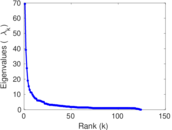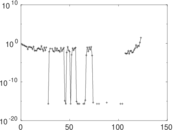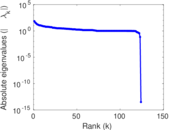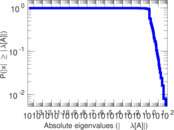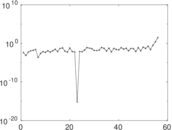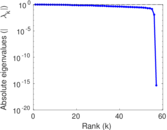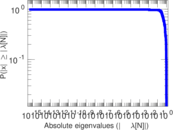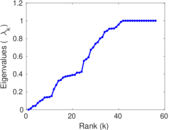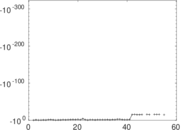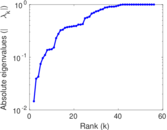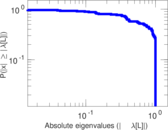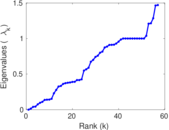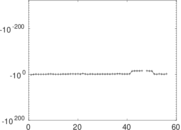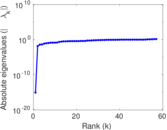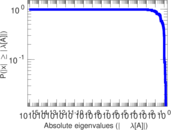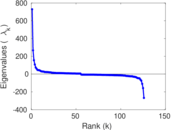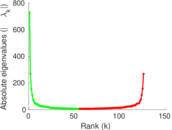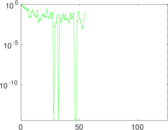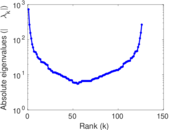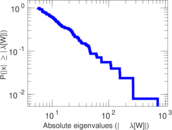# Wiktionary edits (ik)

This is the bipartite edit network of the Inupiaq Wiktionary. It contains users and pages from the Inupiaq Wiktionary, connected by edit events. Each edge represents an edit. The dataset includes the timestamp of each edit.

 Code `mik` Internal name `edit-ikwiktionary` Name Wiktionary edits (ik) Data source http://dumps.wikimedia.org/ AvailabilityDataset is available for download Consistency checkDataset passed all tests Category Authorship network Dataset timestamp 2017-10-20 Node meaning User, article Edge meaning Edit Network formatBipartite, undirected Edge typeUnweighted, multiple edges Temporal dataEdges are annotated with timestamps

## Statistics

 Size n = 741 Left size n1 = 126 Right size n2 = 615 Volume m = 1,873 Unique edge count m̿ = 1,119 Wedge count s = 34,176 Claw count z = 982,289 Cross count x = 24,885,020 Square count q = 19,645 4-Tour count T4 = 296,410 Maximum degree dmax = 444 Maximum left degree d1max = 444 Maximum right degree d2max = 46 Average degree d = 5.055 33 Average left degree d1 = 14.865 1 Average right degree d2 = 3.045 53 Fill p = 0.014 440 6 Average edge multiplicity m̃ = 1.673 82 Size of LCC N = 456 Diameter δ = 15 50-Percentile effective diameter δ0.5 = 5.102 01 90-Percentile effective diameter δ0.9 = 7.937 20 Median distance δM = 6 Mean distance δm = 5.431 25 Gini coefficient G = 0.707 050 Balanced inequality ratio P = 0.217 032 Left balanced inequality ratio P1 = 0.144 154 Right balanced inequality ratio P2 = 0.264 282 Relative edge distribution entropy Her = 0.834 648 Power law exponent γ = 3.123 04 Tail power law exponent γt = 2.131 00 Tail power law exponent with p γ3 = 2.131 00 p-value p = 0.000 00 Left tail power law exponent with p γ3,1 = 1.701 00 Left p-value p1 = 0.867 000 Right tail power law exponent with p γ3,2 = 2.311 00 Right p-value p2 = 0.000 00 Degree assortativity ρ = −0.017 797 1 Degree assortativity p-value pρ = 0.552 031 Spectral norm α = 69.390 2 Algebraic connectivity a = 0.014 564 6 Spectral separation |λ1[A] / λ2[A]| = 1.769 38 Controllability C = 475 Relative controllability Cr = 0.656 985

## Plots

### Fruchterman–Reingold graph drawing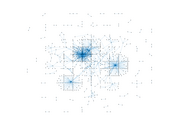### Degree distribution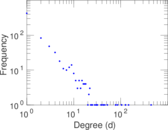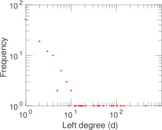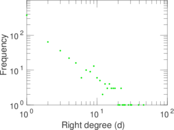### Cumulative degree distribution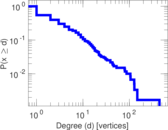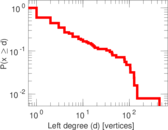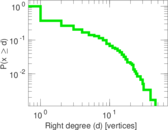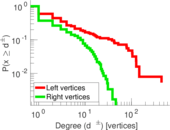### Lorenz curve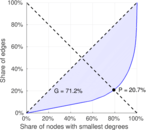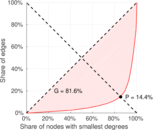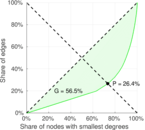### Spectral distribution of the adjacency matrix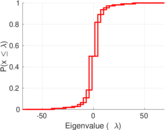### Spectral distribution of the normalized adjacency matrix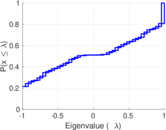### Spectral distribution of the Laplacian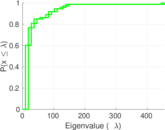### Spectral graph drawing based on the adjacency matrix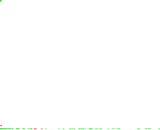### Spectral graph drawing based on the Laplacian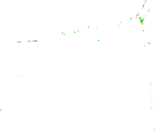### Spectral graph drawing based on the normalized adjacency matrix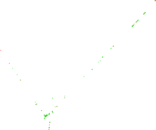### Degree assortativity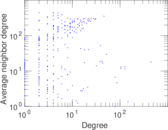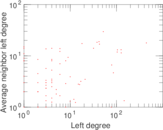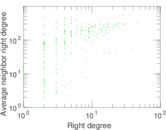### Zipf plot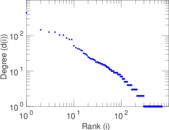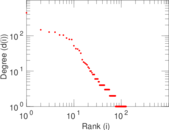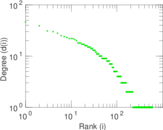### Hop distribution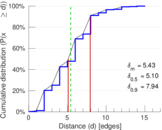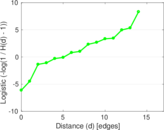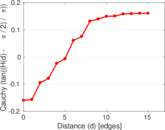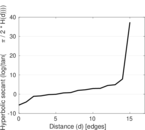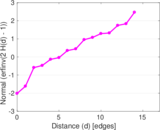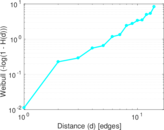### Double Laplacian graph drawing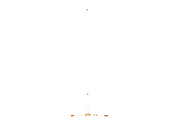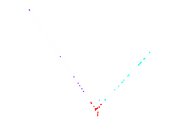### Delaunay graph drawing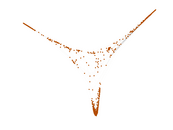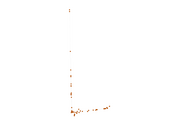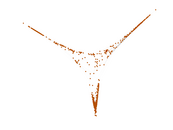### Edge weight/multiplicity distribution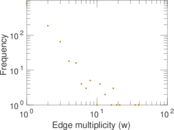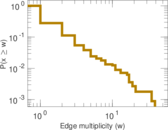### Temporal distribution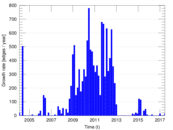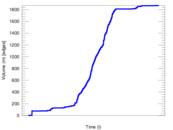### Temporal hop distribution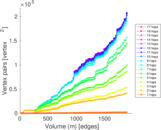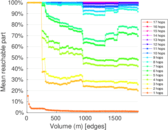### Diameter/density evolution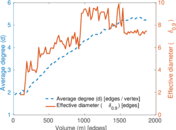### Matrix decompositions plots